Project

# An RMS Detector for a Wideband Voltmeter—Design and Operation

June 25, 2023 by John Woodgate

## This project demonstrates the design and operation of a root-mean-squared (RMS) detector for inclusion in a wideband voltmeter. The RMS detector is useful for measuring certain signal types, including noise, since it provides an indication of the energy in a signal.

In my previous project, I demonstrated a wideband voltmeter that used a peak detector to determine the maximum signal amplitude. The peak detector is suitable for many measurements, however, for some measurements including noise, a root-mean-squared (RMS) detector is necessary. The block diagram of the wideband voltmeter with an RMS detector replacing the original peak detector is shown in Figure 1.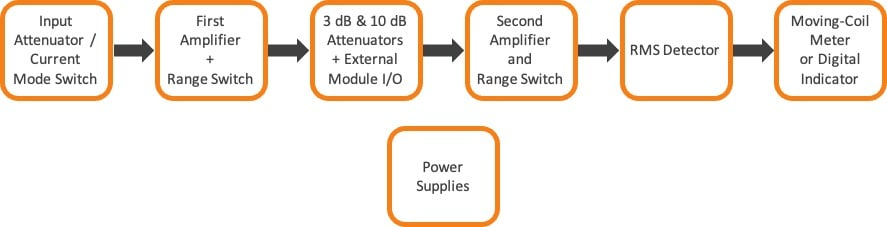### The Math of RMS

Before we dive into the circuits, let’s do a quick refresher on the math. To generate the Root-Mean-Square value of a signal, we need to apply the words in the term from right to left, which is a bit confusing.

First, square the signal (multiply it by itself, not cut off the peaks!). Then calculate the mean value (average value). The mean is normally measured over at least one cycle, but occasionally a half-cycle average is used. Finally, take the square root of the voltage across the capacitor.

Mathematically the RMS calculation is represented as:

$$V_{rms} = \sqrt{mean(V^2)}$$

### Designing an RMS Detector

While it is possible to make an RMS detector from discrete components, carefully matched devices are necessary, and the performance can often be disappointing. An integrated circuit (IC can offer much better performance, especially if laser trimming is applied during manufacture. This is not a low-cost process, so such devices are more costly than, say, general-purpose op amps and comparators.

The Analog Devices AD736 is a true RMS-to-DC converter IC and is available in an affordable version (AD736J) that provides good performance (and in three more costly versions with precision performance). For all versions, the useful bandwidth is limited to 200 kHz, but that is usually quite acceptable for RMS measurements not made by specialized instruments.

The RMS detector circuit schematic is shown in Figure 2 and replaces the circuit of Figure 5 from the original wideband voltmeter project.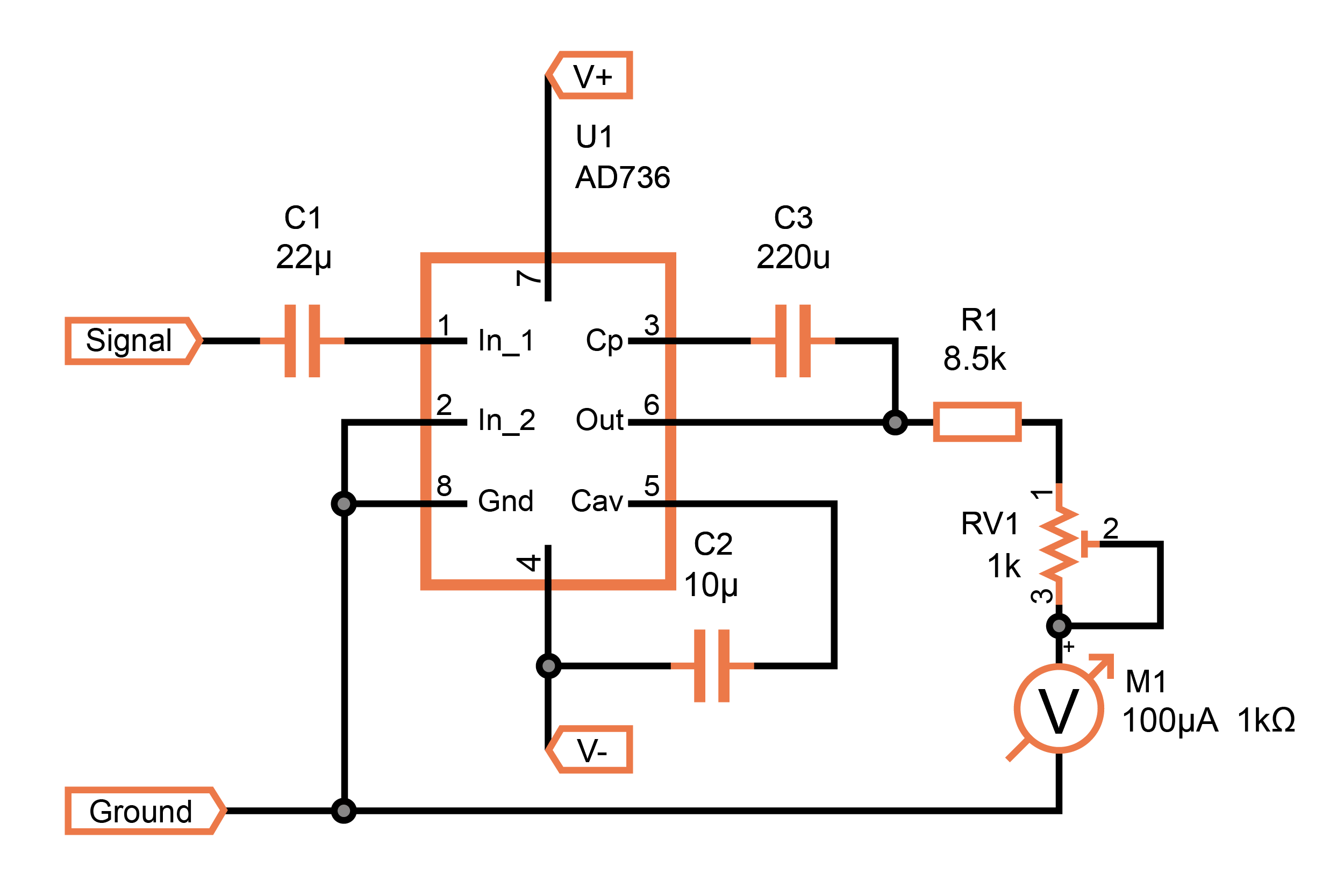##### Figure 2. RMS detector and moving-coil meter circuit

The RMS detector is often used for measuring waveforms that vary rapidly in amplitude, so a digital display is not usable. The choice is between a moving-coil meter and an LED bar graph with a resolution of 1 dB or smaller.

### Frequency Response of the RMS Detector

The technique used in the AD736 and similar devices results in a high-frequency response that varies with signal level. In the present application, this is not a serious problem because the signal level applied to the device can be kept within the range 316 mV to 1 V, except when the voltmeter is set to maximum sensitivity on the 1 mV range.

Figure 3 shows the frequency responses at three input signal levels. The response falls off quite steeply at the band limit.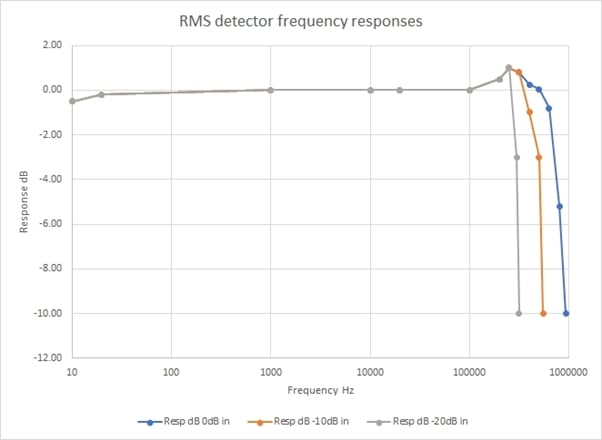### Moving-Coil Meter Error

The error in the reading of the moving-coil meter is an important characteristic. It varies, of course, with the size of the meter because small deflections are difficult to read on a small scale. The results with the instrument I used, which has a 110 mm scale length, are shown in Figure 4.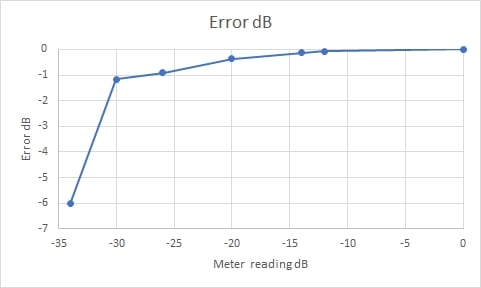##### Figure 4. The error of the moving-coil meter as a function of signal magnitude

Of course, even with this quite large meter, the deflections at -30 dB and -34 dB are very small, and one would not expect to read those levels accurately.

### Operation of the RMS-to-DC Converter

A simplified block diagram of the Analog Devices AD736 is shown in Figure 5.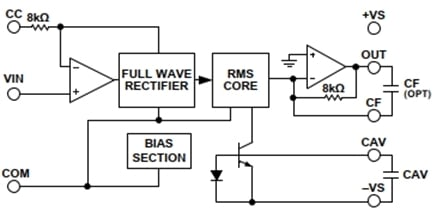##### Figure 5. Block diagram of the AD736. Image used courtesy of Analog Devices

Figure 6 provides additional details of the Analog Devices’ circuit design. The device uses a translinear circuit that is outlined near the center of Figure 6 and labeled ‘RMS TRANSLINEAR CORE. The translinear circuit consists of only bipolar transistors (CMOS could be used in some cases) and current sources. There are no passive components.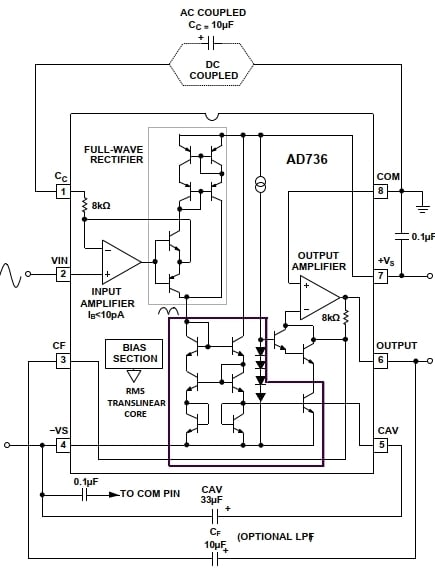##### Figure 6. Internal structure of the AD736. Image used by courtesy of Analog Devices

The data sheet doesn’t divulge all of Analog Devices’ secrets, but we can evaluate the basics of its operation. The squaring and square root operations are carried out by the translinear circuit. For squaring, the signal is presented as a current to a base-emitter junction. The voltage developed across it is proportional to the logarithm of the current. This is just the converse of the more familiar expression that the current is proportional to the exponent of the voltage:

$$I = I_r e^{\frac{qV}{kT}}$$

Taking the logarithm of both sides:

$$\ln(I) = \ln(I_r) + \frac{qV}{kT}$$

The first term on the right, ln(Ir), is very small and $$\frac{q}{kT}$$ is a constant (at a fixed temperature), so the voltage V is proportional to ln(I).

Now if we amplify the voltage by a factor of 2, the result is the log of the square. We apply this to a capacitor CAV, the voltage across which is the average voltage over one cycle.

We apply half this average voltage to a base-emitter junction (effectively applying the log of the square root) and recover the RMS value of the signal as the collector current.

The averaging capacitor, CAV, is charged through the effective resistance of the base-emitter diode, which is inversely proportional to the current through it, so the averaging time increases considerably at low signal levels. However, the range switching of the voltmeter circuit allows for the signal applied to the AD736 to be kept between 316 mV and 1 V, except on the most sensitive range.

The present application uses the low-impedance input of the AD736 at Pin 1 because that accepts a higher maximum input voltage and a wider bandwidth (see Figures 3, 6, and 7 of the datasheet if you want more details). The achievable 200 kHz bandwidth is less than the 1 MHz obtained with the peak detector but is nevertheless more than adequate for many measurements.

### Selecting the Best Measurement Type

It is useful to know the differences in measured values for different waveforms. Table 1 provides a helpful comparison of different signal measurements for five different input waveforms.

##### Table 1. Comparison of Measurement Values for Different Input Signal Types
 Waveform Crest factor Peak RMS Average Sine wave √2 1 1/√2 2/π Square wave 1 1 1 1 Triangle wave √3 1 1/√3 0.5 Noise 1 to 4 up to 4 1 typically 1/√2 Music 1.5 to 10 up to 10 1 typically 1/√2

The crest factor is the ratio of the peak amplitude to the RMS value. The crest factor is a useful number to keep in mind, as it can indicate when peak-clipping of a signal might be occurring. The average detector is the least useful and potentially gives the most misleading results, so there are no plans to provide one for the wideband voltmeter design.

As you can see from this table, there is no simple answer to what is the “best” measurement because it depends on the input signal. Understanding these differences and the operation of the RMS detector presented here can be helpful in designing circuits, making sense of measurement outputs, and troubleshooting circuit problems.• H
hugocoolens June 30, 2023

Dear John, Could you explain how you determined the “Moving-Coil Meter Error”? Normally for that type of voltage meter a class is specified which gives the absolute error value as a percentage of the full scale.

Like.
• E
Elbow Zonderbar June 30, 2023

Interesting article. Thanks. 😊

Like.
• J
JDenenberg June 30, 2023

This is an analog approach to an RMS meter an is interesting. I have also seen/used simple digital RMS meters that randomly sample the waveform (random time intervals) and then use a low cost imbedded processor to calculate the sum-of-squares over a time window. The ones I have used (low cost sound meters) are calculating power levels, but doing a square root to get RMS is a straight forward additional step.

Like.